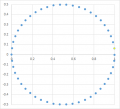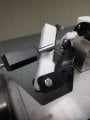# How to calculate projectile locus of a circle where the two motors controlling the "gun" can only move 30° in the X and 30° in the Y?

Joined Sep 14, 2020
29
This could be considered a homework problem, but beyond the math it's for a specific application. There are various shapes I want to make from polar curves. One of the most simple ones is a circle:
r = cos(θ) or
x = cos²(θ) and y = cos(θ)*sin(θ).

Say I want to take increments of theta by 0.0314 to pi. That would make this table:
 θ X Y 0.03 0.9961​ 0.0626​ 0.06 0.9843​ 0.1243​ 0.09 0.9649​ 0.184​ 0.13 0.9382​ 0.2408​ 0.16 0.9046​ 0.2938​ 0.19 0.8646​ 0.3421​ 0.22 0.8189​ 0.3851​ 0.25 0.7681​ 0.422​ 0.28 0.7131​ 0.4523​ 0.31 0.6548​ 0.4754​ 0.35 0.594​ 0.4911​ 0.38 0.5318​ 0.499​ 0.41 0.469​ 0.499​ 0.44 0.4067​ 0.4912​ 0.47 0.3459​ 0.4757​ 0.50 0.2876​ 0.4526​ 0.53 0.2325​ 0.4225​ 0.57 0.1817​ 0.3856​ 0.60 0.1359​ 0.3427​ 0.63 0.0959​ 0.2944​ 0.66 0.0622​ 0.2415​ 0.69 0.0354​ 0.1847​ 0.72 0.0159​ 0.1251​ 0.75 0.004​ 0.0634​ 0.79 0​ 0.0008​ 0.82 0.0038​ -0.0618​ 0.85 0.0155​ -0.1235​ 0.88 0.0348​ -0.1832​ 0.91 0.0614​ -0.2401​ 0.94 0.0949​ -0.2931​ 0.97 0.1348​ -0.3416​ 1.00 0.1805​ -0.3846​ 1.04 0.2312​ -0.4216​ 1.07 0.2861​ -0.452​ 1.10 0.3444​ -0.4752​ 1.13 0.4052​ -0.4909​ 1.16 0.4674​ -0.4989​ 1.19 0.5302​ -0.4991​ 1.22 0.5925​ -0.4914​ 1.26 0.6533​ -0.4759​ 1.29 0.7117​ -0.453​ 1.32 0.7668​ -0.4229​ 1.35 0.8177​ -0.3861​ 1.38 0.8635​ -0.3433​ 1.41 0.9037​ -0.2951​ 1.44 0.9374​ -0.2422​ 1.48 0.9643​ -0.1855​ 1.51 0.9839​ -0.1258​ 1.54 0.9959​ -0.0642​ 1.57 1​ -0.0016​
That makes this locus:Relevant equation, I think:
[width of control surface]*sin(degrees) = linear distance

Say I want to create a 100 foot diameter circle. That means the grid needs to be about 141x141 feet.
I think I need to consider the z-axis. Any suggestions?

If it helps, here is the 30° X and Y constrained apparatus:And this paper might be related, but I could be just overthinking this all: On the locus formed by the maximum heights of projectile motion with air resistance.

Last edited:

Joined Sep 14, 2020
29
For each plane
 Start End 0° 30° 0 50 feet

Or actually, 1° = 1.67 feet. Hmm, how to tie this in...

Joined Sep 14, 2020
29
Last, I think, have to figure out how many steps (integers) can be done by the stepper motor to see how bad of a resolution results:
 Stepper 0​ ? 100 Degrees 0​ 0.9​ 1.8​ 2.7​ 3.6​ 4.5​ 5.4​ 6.3​ 7.2​ 8.1​ 9​ 9.9​ 11​ 12​ 13​ 14​ 14​ 15​ 16​ 17​ 18​ 19​ 20​ 21​ 22​ 23​ 23​ 24​ 25​ 26​ 27​ 28​ 29​ 30​ Distance 0​ 0.03​ 0.06​ 0.09​ 0.12​ 0.15​ 0.18​ 0.21​ 0.24​ 0.27​ 0.30​ 0.33​ 0.36​ 0.39​ 0.42​ 0.45​ 0.48​ 0.51​ 0.54​ 0.57​ 0.60​ 0.63​ 0.66​ 0.69​ 0.72​ 0.75​ 0.78​ 0.81​ 0.84​ 0.87​ 0.90​ 0.93​ 0.96​ 0.99​

This, I think:Side note, I am using the microstepping capability of the motors. It's just I also want the torque to be decent too.

Last edited:

Joined Sep 14, 2020
29
So now it's more of an Arduino question:

Code:
const int dirPin = 2;
const int stepPin = 3;

// fullstep  = 50
// 1/2 step  = 100 → MS1 high
// 1/4 step  = 200 → MS2 high
//      ↓
const int steps = 100;

const int wait = 3;   // milliseconds

void setup()
{
pinMode(stepPin, OUTPUT);
pinMode(dirPin, OUTPUT);
}
void loop()
{
// Set motor direction clockwise
digitalWrite(dirPin, HIGH);  // Forward

int var = 0;
while (var < steps)
{
digitalWrite(stepPin, HIGH);
delay(wait);
digitalWrite(stepPin, LOW);
delay(wait);
var++;
}

// Set motor direction counterclockwise
digitalWrite(dirPin, LOW);

// Backward
var = 0;
while (var < steps)
{
digitalWrite(stepPin, HIGH);
delay(wait);
digitalWrite(stepPin, LOW);
delay(wait);
var++;
}
}
How to translate digitalWrite and delays into steps...

#### ci139

Joined Jul 11, 2016
1,696

? why bother such mess in a first place

? why not just ±90° Z ±?° "X" . . . (( considering you got a stepper motors - so you can achieve any ±2πN by it))

Joined Sep 14, 2020
29
How are Z rotations factored in mathematically?

? why bother such mess in a first place
Sorry, with what?

? why not just ±90° Z ±?° "X" . . . (( considering you got a stepper motors - so you can achieve any ±2πN by it))

#### MrAl

Joined Jun 17, 2014
8,554
How are Z rotations factored in mathematically?

Sorry, with what?

Hi,

I think we already have enough crop circles thank you very muchDo you have to work in 3d then?
If so and you have to rotate in 3d space, look up the rotation matrix for 3 dimensions.

#### ci139

Joined Jul 11, 2016
1,696
How are Z rotations factored in mathematically?
physically + mathematically -- then you work out the best adjustment . . . but such will be an overkill ...
Sorry, with what?
... because you need 2 rotations only instead of 3 (3 would be a bigger mess or the particular 2 that you plan to use right now)
+Plus : what this thing suppose to do AND how precisely/reliably it should operate (? are people health risks involved ? falling objects)
why you use a "cryptic" apparatus of X Y (both horizontal) axis ... instead of the Z (vertical) + "X" (horizontal)

Joined Sep 14, 2020
29
This is a solution that is working:

Code:
const int dirPinX = 2;
const int stepPinX = 3;
const int dirPinY = 4;
const int stepPinY = 5;

const int A = 100;
float deltaH = PI/1000;
float deltaX = 0;
float deltaY = 0;

float h = 0;
float x1 = 0;
float x2 = 0;
float y1 = 0;
float y2 = 0;

void setup() {
Serial.begin(9600);

pinMode(stepPinX, OUTPUT);
pinMode(dirPinX, OUTPUT);
pinMode(stepPinY, OUTPUT);
pinMode(dirPinY, OUTPUT);

int i = 0;
while(i < A/2)
{
digitalWrite(dirPinX, LOW);

digitalWrite(stepPinX, HIGH);
delay(10);
digitalWrite(stepPinX, LOW);
delay(10);
i++;
}
}

void loop()
{

while (h < 2*PI)
{
fx1();
fx2();

fy1();
fy2();

deltaX = deltaX + x2 - x1;
if(abs(deltaX) > 1)
{
stepX();
deltaX = 0;
}

deltaY = deltaY + y2 - y1;
if(abs(deltaY) > 1)
{
stepY();
deltaY = 0;
}

h += deltaH;
}

}

void fx1(){
x1 = A*cos(h)*cos(h);

}

void fx2(){

x2 = A*cos(h+deltaH)*cos(h+deltaH);

}
void fy1(){
y1 = A*cos(h)*sin(h);

}

void fy2(){

y2 = A*cos(h+deltaH)*sin(h+deltaH);

}
void stepX()
{
if(deltaX < 0)  // Set motor direction clockwise
{
digitalWrite(dirPinX, HIGH);
}
else
{
digitalWrite(dirPinX, LOW);
}
digitalWrite(stepPinX, HIGH);
delay(10);
digitalWrite(stepPinX, LOW);
delay(10);
}

void stepY()
{
if(deltaY < 0)  // Set motor direction clockwise
{
digitalWrite(dirPinY, HIGH);
}
else
{
digitalWrite(dirPinY, LOW);
}
digitalWrite(stepPinY, HIGH);
delay(10);
digitalWrite(stepPinY, LOW);
delay(10);
}